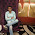### Chicken Nuggets

Heard this problem many times. This problem is everywhere.

You can go to a fast food restaurant to buy chicken nuggets in 6-pack, 9-pack or 20-packs. is there such a number N, such that for all numbers bigger than or equal to N, you can buy that number of chicken nuggets?

Solution:
Highlight the part between the * symbols for the answer.
*Yes. The number is 44

All numbers can be written in the form 3n-1, 3n or 3n+1. Now taking all nos. of the form 3n, all such numbers (except 3) can be formed by combination of 6 and 9.
Now let us take all numbers of the form 3n-1 which are greater than 20, starting with 23, 26, 29.... now again using the same concept like in the last part if we subtract 20(i.e. including a 20 nugget pack) from these numbers, the rest can be formed by combination of 6 and 9 other than 23 which would be the largest such number so far.
Similarly we will take all numbers of the form 3n-2 which are greater than 40, starting with 43, 46, 49.... and we will use the same concept like in the last part, if we subtract 40(i.e. including two 20 nugget packs) from these numbers, the rest can be formed by combination of 6 and 9 other than 43 which would be the largest number and so the answer to the puzzle is 44.*

1.We can get 1 by subtracting 20 and adding two 6's and a nine. Similarly 2 = 20 - 2*9, 3 = 9-6, 4 = 2*9+6 - 20, 5= 20-9-6. So having 2 9's, one 6 and one 20, we will be able to add any natural number. So we must have 2*9+6+20 = 44. So 44 is the answer. We cannot get 43 by a combination of 6,9 and 20